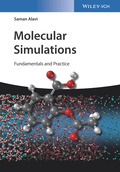Wiley-VCH, Weinheim Molecular SimulationsAls Einführung in die molekulare Modellierung füllt dieses Buch eine Lücke für alle, die die Naturwi.. Product #: 978-3-527-34105-4 Regular price: \$85.62 \$85.62 Auf Lager

# Molecular Simulations

## Fundamentals and Practice1. Auflage Juni 2020
XVI, 326 Seiten, Softcover
150 Abbildungen (30 Farbabbildungen)
Lehrbuch

ISBN: 978-3-527-34105-4
Wiley-VCH, Weinheim

### Kurzbeschreibung

Als Einführung in die molekulare Modellierung füllt dieses Buch eine Lücke für alle, die die Naturwissenschaften auf molekularer Ebene verstehen möchten, aber nicht zu dem Kreis der Experten zählen.

Jetzt kaufen

### Preis: 89,90 €

Preis inkl. MwSt, zzgl. Versand

Provides hands-on knowledge enabling students of and researchers in chemistry, biology, and engineering to perform molecular simulations

This book introduces the fundamentals of molecular simulations for a broad, practice-oriented audience and presents a thorough overview of the underlying concepts. It covers classical mechanics for many-molecule systems as well as force-field models in classical molecular dynamics; introduces probability concepts and statistical mechanics; and analyzes numerous simulation methods, techniques, and applications.

Molecular Simulations: Fundamentals and Practice starts by covering Newton's equations, which form the basis of classical mechanics, then continues on to force-field methods for modelling potential energy surfaces. It gives an account of probability concepts before subsequently introducing readers to statistical and quantum mechanics. In addition to Monte-Carlo methods, which are based on random sampling, the core of the book covers molecular dynamics simulations in detail and shows how to derive critical physical parameters. It finishes by presenting advanced techniques, and gives invaluable advice on how to set up simulations for a diverse range of applications.

-Addresses the current need of students of and researchers in chemistry, biology, and engineering to understand and perform their own molecular simulations
-Covers the nitty-gritty - from Newton's equations and classical mechanics over force-field methods, potential energy surfaces, and probability concepts to statistical and quantum mechanics
-Introduces physical, chemical, and mathematical background knowledge in direct relation with simulation practice
-Highlights deterministic approaches and random sampling (eg: molecular dynamics versus Monte-Carlo methods)
-Contains advanced techniques and practical advice for setting up different simulations to prepare readers entering this exciting field

Molecular Simulations: Fundamentals and Practice is an excellent book benefitting chemist, biologists, engineers as well as materials scientists and those involved in biotechnology.

1. INTRODUCTION
Motivation: Relating Microscopic Molecular Properties to Macroscopic / Thermodynamic Behavior of Bulk Systems
Molecular Dynamics and Monte Carlo Simulations for Modeling Macroscopic Scale Experiment
Scope of the Book and Background Needed

2. CLASSICAL MECHANICS FOR MANY-MOLECULE SYSTEMS
Newton's Equations of Motion for Many-Molecule Systems
Analytical Solution of Newton's Equations for Simple Systems
The Concept of Trajectory and Phase Space
Numerical Solution of Newton's Equations, Finite-Difference Method
The Verlet and Leapfrog Time Progression Algorithms
The Trajectory for a Many-Particle System

3. FORCE FIELD MODELS IN CLASSICAL MOLECULAR SIMULATIONS
Summary of the Quantum Mechanics of Molecular Force Fields
Modeling the Potential Energy Surface of Non-Reactive Systems
Inter- and Intra-Molecular Force Fields
Design and Choice of Force Fields
Calculation of the Force Field Parameters Using Quantum Chemistry, Spectroscopy, and Modeling

4. INTRODUCTION TO PROBABILITY CONCEPTS
Basic Concepts of Probability Theory: Single and Multiple Variable Probabilities, Discrete and Continuous Probabilities, the Central Limit Theorem
Maxwell-Boltzmann Probability Distribution for Molecular Velocity, Speed
Maxwell-Boltzmann Energy Distribution for Single Molecules and Collections of Molecules
Probability Distributions of Large Collections of Molecules
Assigning Molecular Velocities in Molecular Simulations from the Maxwell-Boltzmann Probability Distribution

5. INTRODUCTION TO STATISTICAL MECHANICS
Statistical Mechanics in Classical Mechanics Language
The Concept of Partition Function and Ensembles in Statistical Mechanics:
Canonical (Isothermal Isochoric), Isothermal Isobaric, Grand-Canonical and Other Ensembles
Thermodynamic Properties: Energy, Temperature, Pressure, Entropy, Free Energy, Fluctuations in These Quantities in Microscopic Systems
The Quantum Mechanical Approach to Statistical Mechanics

6. MOLECULAR DYNAMICS (MD) SIMULATIONS 1
Periodic Boundary Conditions: Simulating "Infinite" Bulk Systems with a Finite Number of Molecules
Simulating Bulk Phases, Surfaces, and Nanoparticles;
Short-Range Van Der Waals Forces: Truncation of Potentials
Long-Range Electrostatic Forces: Ewald Summations

7. MOLECULAR DYNAMICS (MD) SIMULATIONS 2
Including the Effect of the Environment in Molecular Simulations: Thermostats and Barostats
Determining Thermodynamic Averages from Molecular Dynamics Trajectories: Multiple Time Origins and Maximizing Sample Size and Statistical Averaging

8. ANALYZING MOLECULAR DYNAMICS SIMULATIONS
Characterizing the Microscopic Structure of Phases
Dynamics of Molecules from MD
Mean-Square Displacements, Velocity Autocorrelations, Diffusion Coefficients

9. MONTE CARLO (MC) SIMULATION METHODS AND APPLICATIONS
Principles of Monte Carlo Methods, Sampling from the Ensemble Probability Distribution
Importance Sampling, Microscopic Reversibility
Advantage and Disadvantages of Monte Carlos Simulations in Comparison to MD Simulations
Simulations in Different Ensembles with MC